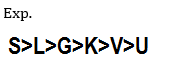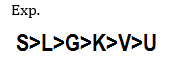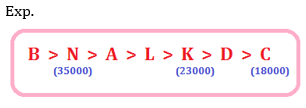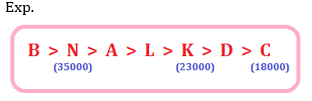# RBI Assistant Pre 2022: Reasoning Ability Quiz-13

## RBI Assistant 2022: Reasoning Ability Quiz

Aspirants have a strong possibility of scoring well in the Reasoning Ability section if they practice quality questions on a regular basis. This section takes the least amount of time if the practice is done every day in a dedicated manner. In this article, we have come up with the RBI Assistant 2022 Reasoning Ability Quiz to help you prepare better. Candidates will be provided with a detailed solution for each question in this RBI Assistant 2022 Reasoning Ability Quiz. This RBI Assistant 2022 Reasoning Ability Quiz includes a variety of questions ranging in difficulty from easy to tough. This RBI Assistant 2022 Reasoning Ability Quiz is totally FREE. This RBI Assistant 2022 Reasoning Ability Quiz has important Reasoning Ability Questions and Answers that will help you improve your exam score. Aspirants must practice this RBI Assistant 2022 Reasoning Ability Quiz in order to be able to answer questions quickly and efficiently in upcoming exams.

Directions (1–5): In the following questions, the symbols @, &, *, # and % are used with the following meaning as illustrated below.
‘P # Q’ means ‘P is smaller than Q’.
‘P @ Q’ means ‘P is smaller than or equal to Q’.
‘P * Q’ means ‘P is greater than or equal to Q’.
‘P % Q’ means ‘P is equal to Q’.
‘P & Q’ means ‘P is greater than Q’.
Now, in each of the following questions assuming the given statements to be true, find which of the two Conclusions I and II given below them is/are definitely true and give your answer accordingly.

1. Statements: I # A ; A & B & C* D@ E; B&F ;E#H ;G&D
Conclusions: I. A&D II. D#H
(a) Only I is true
(b) Only II is true
(c) Neither I nor II is true
(d) Either I or II is true
(e) Both I and II are true

Ans. e
Exp. I. A&D(True) II.D#H(True)

2. Statements: S & R & L; L & M @ N * O % P & Q ; N # T
Conclusions: I. T&Q II. T%Q
(a) Both I and II are true
(b) Only I is true
(c) Only II is true
(d) Either I or II is true
(e) Neither I nor II true

Ans. b
Exp. I.T&Q(True) II.T%Q(False)

3. Statements: V # P ; S % U ; W % R ; P & Q * R @ S % T
Conclusions: I. T @ W II. R* V
(a) Only I is true
(b) Only II is true
(c) Neither I nor II is true
(d) Either I or II is true
(e) Both I and II are true

Ans. c
Exp. I. T@W (False) II.R*V(False)

4. Statements: N* T * R % J & C ; L # J ; R & F # M ; N # S
Conclusions: I. F # S II. N % J
(a) Both I and II are true
(b) Only I is true
(c) Only II is true
(d) Either I or II is true
(e) Neither I nor II true

Ans. b
Exp. I.F#S(True) II. N%J(False)

5. Statements: F # L ; H * C # G ;A & B # C * D @ E % F
Conclusions: I. A @ L II. A & L
(a) Both I and II are true
(b) Only I is true
(c) Only II is true
(d) Either I or II is true
(e) Neither I nor II true

Ans. d
Exp. I. A@L(False) II. A&L(False)

Direction (6-7): Study the following information to answer the given questions
There are six persons-G, K, L, S, U and V. K’s height is greater than only two people. L’s height is more than G. S’s height is more than both L and G. U ‘s height is not more than K. V is not the shortest and not taller than K.
6. Who among the following is the shortest?
(a) K
(b) U
(c) V
(d) L
(e) S

Ans. b7. Who among the following is the second tallest?
(a) K
(b) U
(c) V
(d) L
(e) S

Ans. dDirections (8-10): Study the following information carefully to answer the given questions:
Seven persons – A, B, C, D, K, L and N – are seated in ascending order of their salaries. N earns more than L and D. N earns more than A but he does not earn the highest. A earns more than L. The person who earns the second highest receives a salary of Rs. 35,000 while the third lowest earner receives Rs. 23,000. K earn less than L but more than D. C earns Rs. 18,000. D is not the lowest earner.
8. Who among the following earn(s) more than Rs. 23,000 but less than Rs. 35,000?
(a) Only A
(b) Only L
(c) A and L
(d) K and L
(e) A and N

Ans. c9. Who among the following may earn Rs. 21,000?
(a) K
(b) D
(c) L
(d) C
(e) N

Ans. b10. Who among the following earns Rs. 35,000?
(a) A
(b) L
(c) B
(d) K
(e) N

Ans. e#### Aatma Nirbhar Reasoning Topic Wise Online Test Series

RBI Assistant Prelims Online Test Series 2022

Recommended PDF’s for:

### Preparation Kit PDF

#### Most important PDF’s for Bank, SSC, Railway and Other Government Exam : Download PDF Now

AATMA-NIRBHAR Series- Static GK/Awareness Practice Ebook PDF Get PDF here
The Banking Awareness 500 MCQs E-book| Bilingual (Hindi + English) Get PDF here
AATMA-NIRBHAR Series- Banking Awareness Practice Ebook PDF Get PDF here
Computer Awareness Capsule 2.O Get PDF here
AATMA-NIRBHAR Series Quantitative Aptitude Topic-Wise PDF 2020 Get PDF here
Memory Based Puzzle E-book | 2016-19 Exams Covered Get PDF here
Caselet Data Interpretation 200 Questions Get PDF here
Puzzle & Seating Arrangement E-Book for BANK PO MAINS (Vol-1) Get PDF here
3